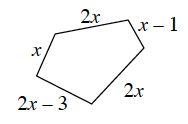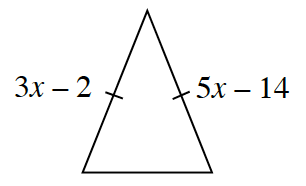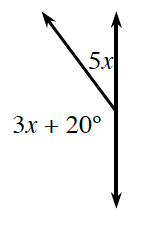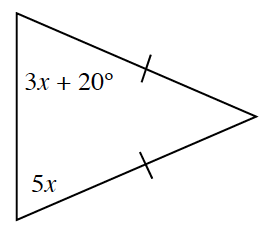### Home > INT2 > Chapter 5 > Lesson 5.1.1 > Problem5-6

5-6.

For each diagram below, set up an equation and solve for $x$.

1. $\text{Perimeter}=76\ \text{units}$Use the equation $2x+x+(2x-3)+2x+(x-1)=76$ to solve for $x$.

1.$3x-2=5x-14$

1.The two angles are supplementary angles.

1.